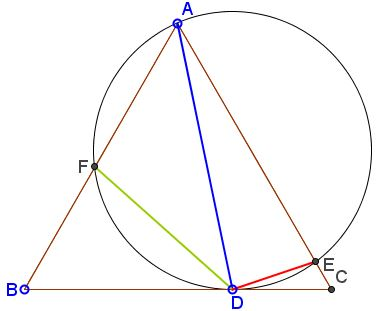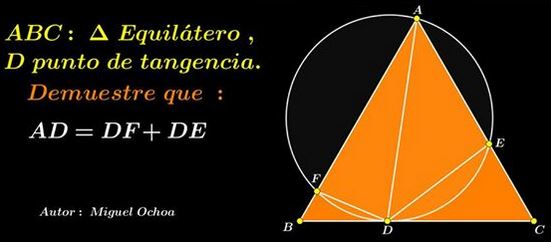# Miguel Ochoa's van Schooten Like Theorem

### What Might This Be About?

10 May 2015, Created with GeoGebra

### Problem

In $\Delta ABC,$ circle $(O)$ through $A$ and tangent to $BC$ in $D$ cuts $AB$ and $AC$ in $E$ and $F,$ respectively.Prove that $AD = DE + DF.$

In the notations I follow the original formulation:### Proof 1

Rotate $A,B,C,F$ $60^{\circ}$ counterclockwise around $D$ into positions $A',B',C',F',$ respectively.Since $\angle EDF=120^{\circ},$ $F'$ is collinear with $D$ and $E$ so that

\begin{align} EF'&=DE+DF'\\ &=DE+DF. \end{align}

I am going to show that

1. $BC'=AD,$

2. $BC'\parallel EF'.$

Since, obviously, $A'B'\parallel AC,$ this will furnish the proof of the statement.

$CC'=CD,$ implying $BD=AC'.$ Also, $\angle BDC=\angle AC'D=120^{\circ}.$ It follows that triangles $BC'D$ and ADC' are equal, so that, in particular, $BC'=AD.$ In addition, $\angle C'BD=\angle DAC'.$ But $\angle DAC'=\angle DAC=\angle CDE,$ the former being an inscribed angle subtended by the arc $DE,$ the latter is defined by the chord $DE$ and the tangent at $D.$ Thus, $\angle C'BD=\angle EDC,$ making $BC'\parallel DE$ and, hence, $BC'\parallel EF'.$

### Proof 2

Without loss of generality, set $AB=1,$ $BD=k$ and $CD=1-k,$ where $k\in(0,1).$ By the Power of a Point Theorem, $BF\cdot AB=BD^{2},$ implying $BF=k^{2}$ and $AF=1-k^2.$ By Stewart's theorem in $\Delta ABD$ and the cevian $DF,$

$FD^{2}\cdot AB+BF\cdot AF\cdot AB=BD^{2}\cdot AF+AD^{2}\cdot BF.$

In other words,

$FD^{2}\cdot AB+k^{2}(1-k^{2})=k^{2}(1-k^{2})+k^{2}AD^{2},$

i.e., $FD=kAD.$ Similarly, $DE=(1-k)AD.$ Adding up finalize the proof.

### Acknowledgment

The above statement has been posted by Miguel Ochoa Sanchez at the CutTheKnotMath facebook page. The second proof is by Leo Giugiuc.

I placed proofs by Josué García Piscoya and Gobbalipur Jayanth on separate pages.

• Angle Trisectors on Circumcircle
• Equilateral Triangles On Sides of a Parallelogram
• Pompeiu's Theorem
• Pairs of Areas in Equilateral Triangle
• The Eutrigon Theorem
• Equilateral Triangle in Equilateral Triangle
• Seven Problems in Equilateral Triangle
• Spiral Similarity Leads to Equilateral Triangle
• Parallelogram and Four Equilateral Triangles
• A Pedal Property in Equilateral Triangle
• Two Conditions for a Triangle to Be Equilateral
• Incircle in Equilateral Triangle
• When Is Triangle Equilateral: Marian Dinca's Criterion
• Barycenter of Cevian Triangle
• Excircle in Equilateral Triangle
• Converse Construction in Pompeiu's Theorem
• Wonderful Trigonometry In Equilateral Triangle
• 60o Angle And Importance of Being The Other End of a Diameter
• One More Property of Equilateral Triangles
• Van Khea's Quickie
• Equilateral Triangle from Three Centroids
•